10.3. Models of the Hydrogen Molecule

As the temperature is reduced, the electrons of the hydrogen atoms move to lower energy levels (come close to the protons). Their bond with the protons becomes stronger, and the conditions appear for the connection into a unified structure of two protons and two electrons. But prior to it the formation of an intermediate structure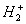is possible , which is called a molecular ion of hydrogen. The structure of such ion is given in Fig. 52 , . Let us pay attention to the formation of its spin.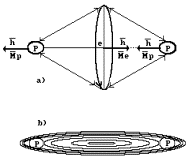Fig. 52. Diagram of molecular ion: a) originates from our theory; b) originates from Schroedinger’s equation

As in general vectors of angular momentum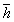and magnetic moment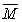coincide in their direction and as the like magnetic poles of the electron and the first proton are directed to meet each other, vectors of their angular momentumare directed to meet each other, that’s why their sum is equal to zero. Vector of angular momentum of the second proton remains uncompensated, that’s why spin of molecular ion of hydrogenis equal to Planck’s constant. The directions of vectors of magnet moments of both protons coincide, that’s why magnet moment of ion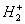is reduced as compared with magnet moment of the hydrogen atom by a value of magnet movement of the second proton and becomes equal  to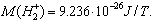Molecular ion of hydrogen is unstable formation as well, because its second proton has weaker connection with the electron and, therefore, it has a stock of free electromagnetic lines in order to be connected with free electron, and this phenomenon does not take place. Hydrogen molecule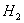is formed (Fig. 53). Magnetic and electrical lines of force interact with each other, that’s why such structure is chemically inactive at usual temperature.

Two protons and two electrons, as it is accepted in modern chemistry, form the structure with rather strong bond equal to 436 kJ/mol. In calculation for one molecule it will be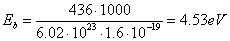,                                              (246)

and for one atom – 2.26 eV.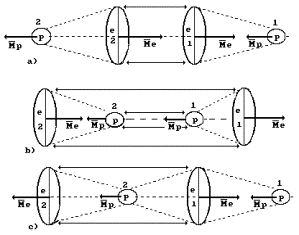Fig.  53. Diagram of the hydrogen molecule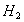: a), b) – orthohydrogen, c) – parahydrogen

Let us pay attention to an important thing. According to the existing notions, the hydrogen molecule can have two structures. In the structure of orthohydrogen, the directions of vectors of magnetic moments of the protons are turned to one direction, and in the structure of parahydrogen to opposite directions . But we have already shown that the value of magnetic moment of the proton is by a factor of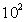less than the value of magnetic moment of the electron, that’s why the hydrogen molecule classification adopted in modern chemistry should be determined not by magnetic moment of the proton, but by magnetic moment of the electron. If this peculiarity is taken into consideration, the hydrogen molecule will have the following difference in its structure.

In Fig.  53, a, b, the directions of vectors of magnetic moments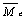of both electrons coincide; it means that the directions of vectors of their angular momentum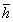coincide. Let us call the structure orthohydrogen. In Fig. 53, c the above-mentioned vectors are opposite, that’s why let us call this structure parahydrogen .

Let us pay attention to the logical actions of the Nature connected with orthohydrogen structure formation (Fig. 53, a). Electro static forces of mutual attraction of the first electron and the first proton are balanced by magnetic forces of these particles turned to opposite directions. That’s why vectors `and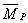of their magnetic moments are turned to opposite directions. Electrostatic forces of repulsion existing between the first electron and the second one are balanced by magnetic forces converging them, that’s why the direction of vectors `of their magnetic moments coincide. In order to compensate for electrostatic forces of mutual attraction of the second electron and the second proton, it is necessary to make magnetic forces of these particles opposite directed ones. This action is reflected in opposite directed vectorsandof magnetic moments of the second proton and the second electron.

One more variant of an arrangement of orthohydrogen molecule is shown in Fig. 53, b. The principle of formation of this molecule is the same. Vectors of magnetic moments of the electrons and the protons are directed in such a way that if electric forces bring the particles nearer each other, magnetic forces should move away them from each other. As a result, an equilibrium is established between them. Stability of structure formed in such a way depends on binding energies between its elements. As magnetic movements of the electrons are by a factor ofgreater than magnetic moments of the protons ,electromagnetic forces of the first structure (Fig. 53, a) keep its elements together stronger than in the structure shown in Fig. 53, b, that’s why there is every reason to believe that the first structure of orthohydrogen is more stable than the second one.

Let us retrace logical actions of the Nature during the formation of a molecule of parahydrogen (Fig. 53, c). The logic of bond formation between the first electron and the first proton remains the same. The forces of mutual attraction of the first electron and the second proton are balanced by their opposite directed magnetic forces.

It is easy to see that the distances between the second proton and the electrons are less than between the first electron and the second one; it means that electrostatic forces of attraction of these electrons to the second proton will be greater than electrostatic forces of repulsion acting between the first electron and the second electron. Electrostatic forces of mutual attraction of the first electron and the second proton are balanced by opposite directed magnetic forces. As they are by a factor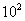of  greater in the electrons than in the protons, vector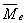of magnetic moment of the second electron should be directed opposite to magnetic moment vector as it is shown in Fig. 53, c.

It is high time to analyze the existing notions concerning the chemical bond between atoms in the molecules. They are described by several theories , , , .

Ion theory of bond describes the bonds established between unlike charged ions. On these grounds the bond between the first proton and the first electron as well as between the second electron and the second proton in the molecules of orthohydrogen (Fig. 53, a) is considered to be an ion bond.

In accordance with the covalent bond theory, it is formed by two non-coupled electrons of tow atoms. Such bond can be observed between the first electron and the second one of the orthohydrogen molecule (Fig. 53, a). The reason why this bond has been called “covalent bond” is not clear for us, we’ll call the bond between the electrons simply the electron bond, between the protons – the proton bond, and the bond between the electron and the proton will be called the electron and proton bond.

The hydrogen bond theory describes chemical bonds realized by the hydrogen atom. The diagram of the hydrogen atom (Fig. 50) shows that the hydrogen atom is an ideal link between the atoms. This bond is formed both by Coulomb’s forces of the proton and the electron and their magnetic forces.

It is known that there is a phenomenon of electron capture by the proton and neutron formation with the emission of the neutrino as the modern physicists think. Let us consider the realization of this  phenomenon on the examples of the formation of the structures of the atom and the hydrogen molecule. It is clear that the situation is possible when vectors of magnetic moments (Figs 50, 51) of the proton and the electron coincide. The electrostatic and magnetic forces will attract the electron and the proton to each other, and the proton will absorb the electron. In order that the proton becomes the neutron, it should absorb more than two electrons. We have already noted that this process is realized during plasma electrolysis of water as the Japanese scientists T. Ohmori and T. Mizuno have found out . The remainder of the third electron is called the neutrino by modern physicists and chemists. As it has no charge, it is an evasive particle. We have the possibility to suppose (i.e. to formulate another hypothesis) that a part of the third electron is not absorbed by the proton, it dissipates as a substance being called the ether .

10.4. Structure of the Helium Nucleus and Atom

As it is known, the helium atom has two electrons. We have already described the method of determination of binding energy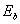of the first electron with the atomic nucleus, which corresponds to its first energy level. It is equal to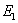=13.48 eV. Taking into consideration of ionization of this electron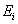=24.587 eV and using spectroscopy law (212), we’ll get the necessary data (Table 30).

Table 30. Spectrum of the first electron of the helium atom

 Volume n 2 3 4 5 6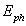(exper.) eV 21.22 23.09 23.74 24.04 24.21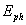(theor.) eV 21.22 23.09 23.74 24.05 24.21(theor.) eV 3.37 1.5 0.84 0.54 0.37

Thus, if the electron of the hydrogen atom interacting with the nucleus is at a distance, which 100fold exceeds the size of the electron itself , it is interesting to know the first electron of the helium atom interacts with the nucleus.

The reply to this question is given by binding energy (3.37 eV) of the first electron with the nucleus when it is on the second energy level (Table 30). In its quantity, this energy resembles binding energy (3.40 eV) of the electron of the hydrogen atom with the nucleus , which consists of one proton only when it is on the second energy level (Table 29). It is clear that at such binding energy with the nucleus the first electron cannot interact with two protons of the nucleus simultaneously as the second electron of the helium atom does having binding energy with the nucleus corresponding to the first energy level, which is equal to 13.6×4=54.40 eV. It appears from this that the first electron of the helium atom interacts only with one proton of its nucleus. It is confirmed by the structure of the nucleus of the helium atom (Fig. 22). Both protons  of the nucleus are arranged at two ends of the nucleus with linear structure. Each electron interacts with its own proton (Fig. 54) .

Let us recollect the spectroscopy analysis results, from which it is clear that all electrons lack orbital motion energy and, consequently, the orbital motion itself. The electron in the atom has only rotational energy relatively its axis, it being the main unexpected result. It makes us think that the electron interacts with the nucleus as a bar magnet, i.e. with its axis of rotation. Thus, the fact of interaction of the first electron of the helium atom by the axis of rotation with its nucleus from the spectroscopy law and is confirmed by a value of binding energy (3.37 eV) with the nucleus when it is on the second energy level.

1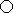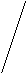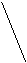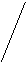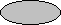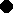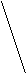N

2

Fig. 54. Model of the nucleus and the atom of helium: N is the nucleus of the atom, 1,2 are the numbers of the electrons

It is necessary to explain the notion “axis of interaction”. It is an imaginary line, which passes through the magnetic poles of the electron or the proton. It connects geometrical centers of the proton or the electron or two electrons when they interact. Magnetic forces act along this line. They provide interaction of these particles and together with Coulomb forces form the conditions for stability of such formation.

When determining the diagram of interaction of the second electron with the nucleus of the helium atom one should take into account that magnetic moment of the helium atom is equal to zero. It means that vectors of magnetic moments and vectors of their angular moment are directed along one straight line in opposite directions. The models of the helium atom with series of energy transitions of the first electron is such as it is given in Fig. 55, c.

It is high time to speak about the notion “orbital motion of electron”. Now this notion does not reflect the essence, which is understood, that’s why it is necessary to introduce a new notion, which should reflect the process of the discovered motion of the electron in the atom more exactly.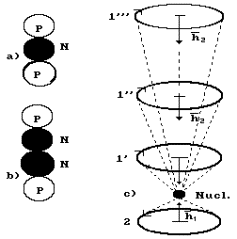Fig. 55. Model of the helium nucleus and the atom with energy transitions of the first electron: a) diagram of the model of the isotope nucleus of the helium atom; b) diagram of the model of the atomic nucleus of the helium atom; c) model of the atom: 1,2 are the numbers of the electrons; N is the atomic nucleus; 1’, 1’’, 1’” are the energy levels of the first electron.

Let us recollect that the chemists embarrassed by the fact that Schroedinger’s equation does not give the opportunity to determine the exact position of the electron in the atom and specifies probability, with which it is possible to determine the position of the electron in this or that field of the atom, have decided to substitute the notion “orbital” for “orbit” implying something uncertain .

Now we see (Fig. 55, c) that during absorption of the photon the electron moves relatively to the nucleus along its axis of rotation, i.e. axis of symmetry, passing from one energy level to another one. That’s why in the future we’ll not use the notion “orbit”, we’ll use the notion “energy level”, or simply “level”. As the axis of rotation of the electron during the interaction with the nucleus can deviate, i.e. it can make precession motion, it is possible to call the movement of the electron in the atom a precession one.

Let us consider the electron charge. It is known that free electrons have one and the same charge. The question arises: is this charge changed during energy transitions of the electron in the atom? There is every reason to believe that it is changed. Let us pay attention to the interaction of the first electron and the second electron in the helium atom. Magnetic force repulsing the second electron from the nucleus, is directed along the axis, which connects their centers, and the electric forces, which exist between the electrons, bring them closer to each other. Regularity of the change of these forces determines regularity of the change of binding energy (213) of the electrons with the nucleus.

A very important and interesting moment has come. As each electron of the helium atom interacts only with its proton, their binding energies with each proton should be equal. But the experiment gives another result. Let us copy out binding energies of the electron of the hydrogen atom with the proton, which correspond to n energy levels, from Table 27 and binding energies of the second electron of the helium atom with its nucleus from Table 10. Let us copy out binding energies with the atomic nucleus of the first electron of the helium atom (Tabl. 13) and put everything in Table 31.

As it is clear, the electron of the hydrogen atom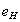and the first electron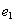of the helium atom have practically equal binding energies with the atomic nuclei at the corresponding energy levels. Binding energy of the second electron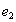of the helium atom with its nucleus corresponding to the first energy level is fourfold greater than the corresponding binding energy of the hydrogen atom, that’s why all other values of binding energies of the electron, which are equal to the corresponding binding energies of the first electron of the helium atom and the electron of the hydrogen atom, are shifted.

Table 31. Binding energiesof the electron of the hydrogen atom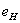and the first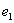and the second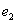of the electrons of the helium atom He with the nuclei

 N 1 2 3 4 5 6 7 8 913.6 3.4 1.51 0.85 0.54 0.38 0.28 0.21 0.17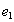- 3.37 1.5 0.85 0.55 0.38 0.28 0.22 0.1754.4 13.6 6.04 3.4 2.18 1.51 1.1 0.85 0.67

Let us pay attention to the fact that within the framework of the permissible error binding energies of the electrons of the helium atom (Table 31) corresponding to the first energy level (n=1) are calculated according to the formula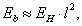(247)

where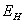is ionization energy of the hydrogen atom, l is the number of the electron in the atom corresponding to the number of the potential of its ionization.

Then the formula for the calculation of binding energy of any electron of the helium atom corresponding to any energy level will be as follows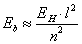(248)

The coincidence of the calculation results according to this formula with the experimental results given in Table 31 proves correctness of the directions of the investigations.

When binding energy of the second electron of the helium atom with the nucleus at the moment of its stay on the third energy level is determined, the formula (248) will be written in such a way: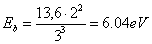.                                                           (249)

A question arises: why does each electron in the helium atom interacts independently from each other with separate protons of the atomic nucleus, and binding energies with the nucleus are different? The answer will be as follows.

In reality when both electrons are in the atom, and each of them interacts with its proton in the nucleus, their binding energies with the nucleus are similar or almost similar. When one electron is removed from the atom, the remaining electron (the second electron) begins to interact with two protons of the nucleus, not with one. Force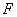of interaction between the electron and the nucleus, which has two protons, will be written in the following way: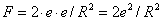.                                                            (250)

If we multiply the right part by radius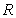, which determines the distance between the electron and the atomic nucleus, we’ll get the value of binding energy of the second electron with the nucleus of helium atom.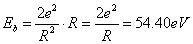.                                                      (251)

It appears from this that if the first electron of the helium atom returns to the atom, binding energy of the nucleus of the second electron will be reduced and will be equal to binding energy with the same nucleus of the first electron of the helium atom.

This conclusion is very important for chemistry. Both electrons of the helium atom have equal probability of formation of the connections with the electrons of other atoms, because they have equal binding energies with the nucleus on all energy levels. Later on we’ll see the same regularity of formation of binding energies with the atoms of the symmetrical structure of the nuclei.

10.5. Structure of the Lithium Atom

We have already shown that the majority of the atomic nuclei of lithium have 4 neutrons and three protons (Fig. 23, a). All the protons have free magnetic poles for the connection with the magnetic poles of the electrons when an atom is formed (Fig. 56).

The connection is established by means of interaction of the unlike electric fields of the protons and the electrons, which bring them close to each other, and the like magnetic poles, which restrict this approach. It comes out that each electron interacts only with one proton of the atomic nucleus (Fig. 56).

The analysis of the diagram of Fig. 56 shows that the electrons, which are arranged symmetrically, will have similar binding energies with the nucleus. The electron, which is arranged to the right of the nucleus, will be influenced by electrostatic forces of repulsion of two other electrons, that’s why it will be situated farther from the nucleus, and its binding energy with the nucleus will be the smallest one. That’s why we confirm that first number to this electron.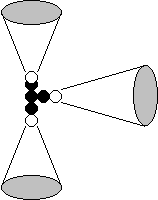2

1

N

3

Fig. 56. Diagram of the models of the nucleus and the atom of lithium: N is the atomic nucleus; 1,2,3 are the numbers of the electrons

Let’s write binding energies of the first electron of the lithium atom with its nucleus out of Table 15 and of the second one out of Table 14. We’ll take binding energies with the nucleus of the lithium atom of the third electron out of Table 8.  Let us make a consolidated Table of binding energies of the electrons of the lithium atoms with its nucleus (Table 32).

Table 32. Binding energies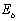of the electron of the hydrogen atom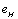and the first, the second and the third electrons of the lithium atom Li with the nucleus

 n 1 2 3 4 5 6 7 8 9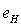13.6 3.40 1.51 0.85 0.54 0.38 0.28 0.21 0.17 1 14.06 3.51 1.56 0.88 0.56 0.39 0.29 0.22 0.17 2 54.16 13.54 6.02 3.38 2.17 1.50 1.10 0.85 0.67 3 122.5 30.6 13.6 7.65 4.90 3.40 2.50 1.91 1.51 n 10 11 12 13 14 15 16 17 18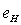0.14 0.11 0.09 0.08 0.07 0.06 0.05 0.05 0.04 1 0.14 0.12 0.10 0.08 0.06 0.06 0.05 0.05 0.04 2 0.54 0.45 0.38 0.32 0.28 0.24 0.21 0.19 0.17 3 1.23 1.01 0.85 0.72 0.63 0.54 0.48 0.42 0.38

If we analyze Table 32, we see proximity of binding energies of the electron of the hydrogen atom and the first electron of the lithium atom on the first, the second and the third energy levels and almost complete coincidence on all the rest ones. It is one of the evidences of the fact that the first electron of the lithium atom interacts with one proton of the nucleus of its atom.

It is not difficult to see that if one (the third) electron remains in the lithium atom, it will begin to interact with three protons at once, and its binding energy with the nucleus, which corresponds to the first energy level, will be determined according to the formula (248).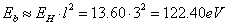,                                               (252)

It coincides with the values of this energy in Table 32 and confirms our hypothesis in relation to the fact that if one electron remains in the atom, it interacts simultaneously with all protons of the nucleus.

Let us calculate binding energy of the third electron of the lithium atom with the nucleus according to the formula (248) when it is on the fifth energy level.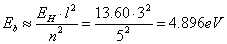(253)

As it is clear, this value matches similar binding energy of the third electron of the lithium atom with the nucleus when it is on the fifth energy level (Table 32). As the lithium atom with one electron is the hydrogen-like atom, let us calculate binding energy of the second electron of this atom with the nucleus to be convinced when it is on the seventh energy level.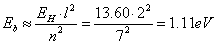.                                                     (254)

This result matches binding energy of the second electron of the lithium atom when it is on the seventh energy level (Table 32). If we managed to measure binding energy of two remaining electrons of the lithium atom with the nucleus without removal of the first electron from it, it would appear that all three electrons have equal binding energies with the nucleus. But it is hardly possible to carry out such an experiment at this stage of scientific research. But we have already given a hypothetical explanation of this phenomenon.

The coincidence of the calculation results according to the formulas (247) and (248) with the experimental results given in Table 32 proves viability of this explanation.

It is easy to imagine that various values of binding energies of various electrons of the lithium atom (Table 32), which correspond to the first energy level (n=1) are obtained due to the fact that after removal of the first electron from the atom the released proton begins to interact with the second electron increasing its binding energy to a value, which is near to binding energy of the second electron of the helium atom  (Table 32).

When the second electron is removed from the atom, two free protons are in the nucleus, which begin to interact with the remaining third electron increasing its binding energy with the nucleus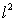fold.

If we begin to return consequently all electrons to the atom, the number of the protons, which have interacted earlier with one electron, begins to be reduced. Binding energy of this electron will be reduced to the value, which is approximately equal to binding energy with the electron nucleus of the hydrogen atom.

The Foundations of Physchemistry of Microworld

Ó2003 Kanarev Ph. M.

Internet Version - http://book.physchemistry.innoplaza.net

<< Back to Physchemistry Book Index